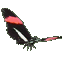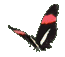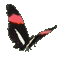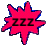Math Help ,    Slope = Rate of Change ,    Line Graph Game Tips :

- The slope (average rate of change) from a point P(-2,-3) to a point Q(1,4)
is calculated as slope = (rise)divided by(run) = (change in y)/(change in x) = (4-(-3))/(1-(-2)) = (7)/(3).

- The rate of change in a relation such as y=mx and its ordered pairs (x,y)
can be measured by the ratio = (change in y)/(change in x) = m = slope.
Generally, the idea is that the average rate = (y2-y1)/(x2-x1) = m = slope.

- Your Game Score is reduced by the number of butterfly hits.

- To slow the game speed repeat tap/click on the word Slider.
- To increase the game speed repeat tap/click on the word Math.
- Speed can also be adjusted with a keyboard's - and + keys.

- Refresh/Reload the web page to restart the game.